NCERT Exemplar Class 8 Maths Solutions for Chapter 9 - Comparing Quantities

NCERT Exemplar Class 8 Maths Solutions for Chapter 9 - PDF Download Free Download

NCERT Exemplar Class 8 Maths Chapter 9 Comparing Quantities, is provided here in PDF format. These exemplars are designed by the subject experts as per the CBSE syllabus(2018-2019) followed by the schools affiliated to Central Board of Secondary Education.

In this chapter, the students will learn about different types of quantities and how to convert one form of quantity into another form. Solve questions related to this topic from the NCERT exemplar to understand comparing quantities in a better and easy way.

Class 8 Maths NCERT Exemplar For Comparing Quantities

To understand the concepts present in each chapter, solve NCERT exemplars for class 8 for Maths as well as Science subject, on all the topics covered under syllabus. Go through the topics based on which exemplars are given for chapter 9.

• Basics of ratios and percentage
• Increase and decrease in the percentage, discount percentage
• Calculate profit and loss
• Tax, compound interest and its formula
• Applications based on compound interest

Students are also provided herewith exemplar books, NCERT solutions for 8th standard Maths and question papers in BYJU’S, as reference tools to use while preparing for exams. Solve sample papers and previous year question papers as it gives an idea of types of questions asked in the board exam from chapter comparing quantities.

Visit the downloadable link to get the free PDF of chapter 9 exemplar.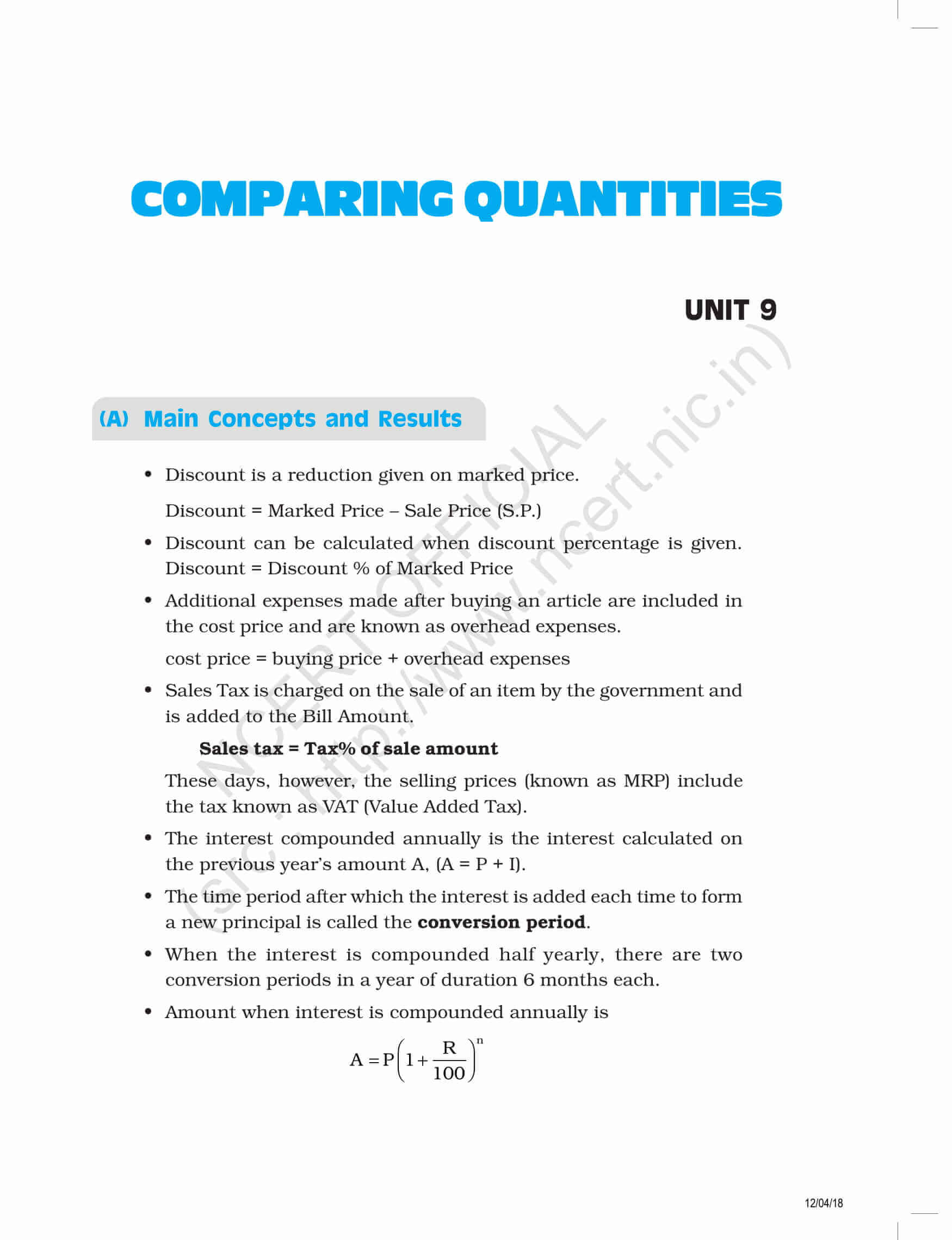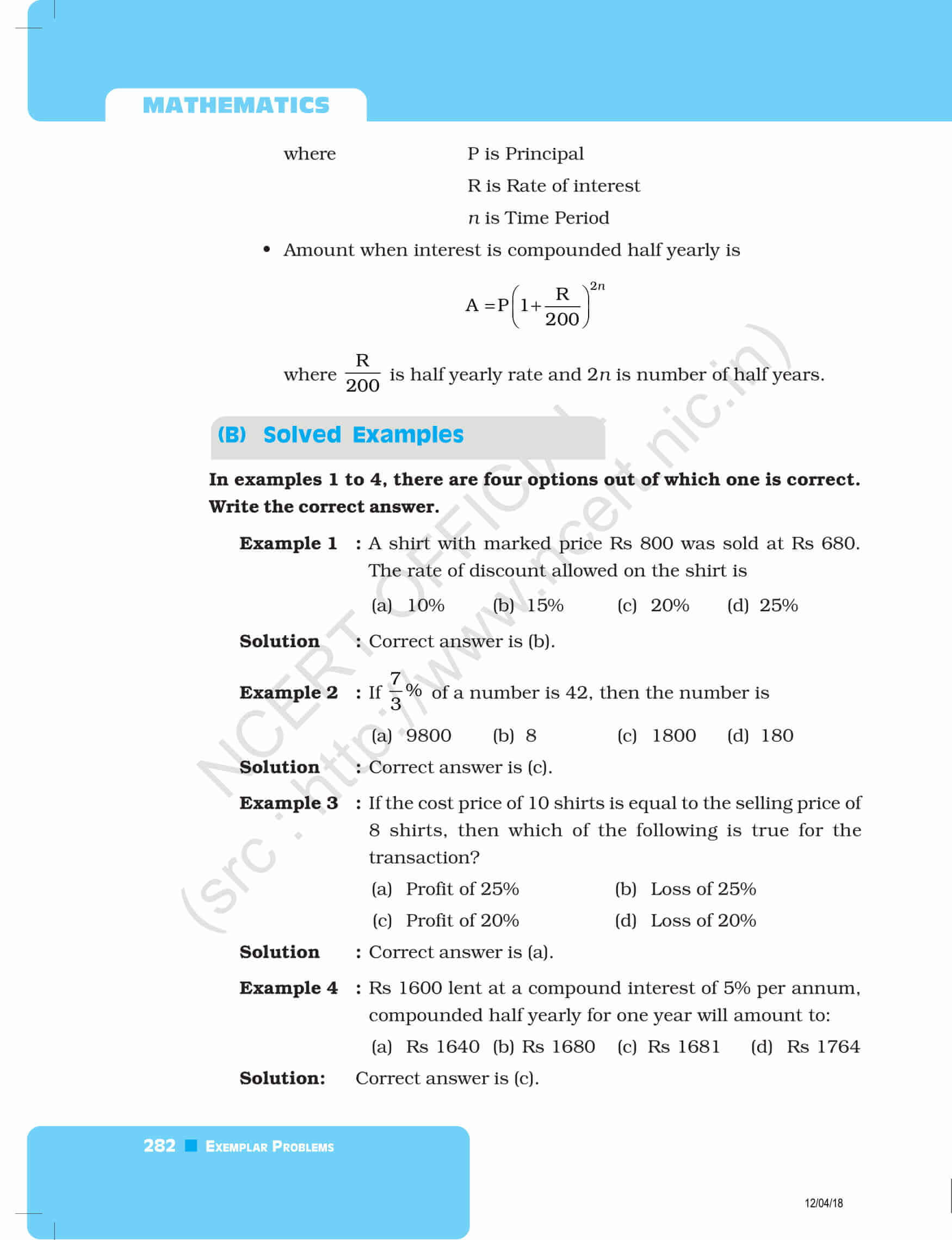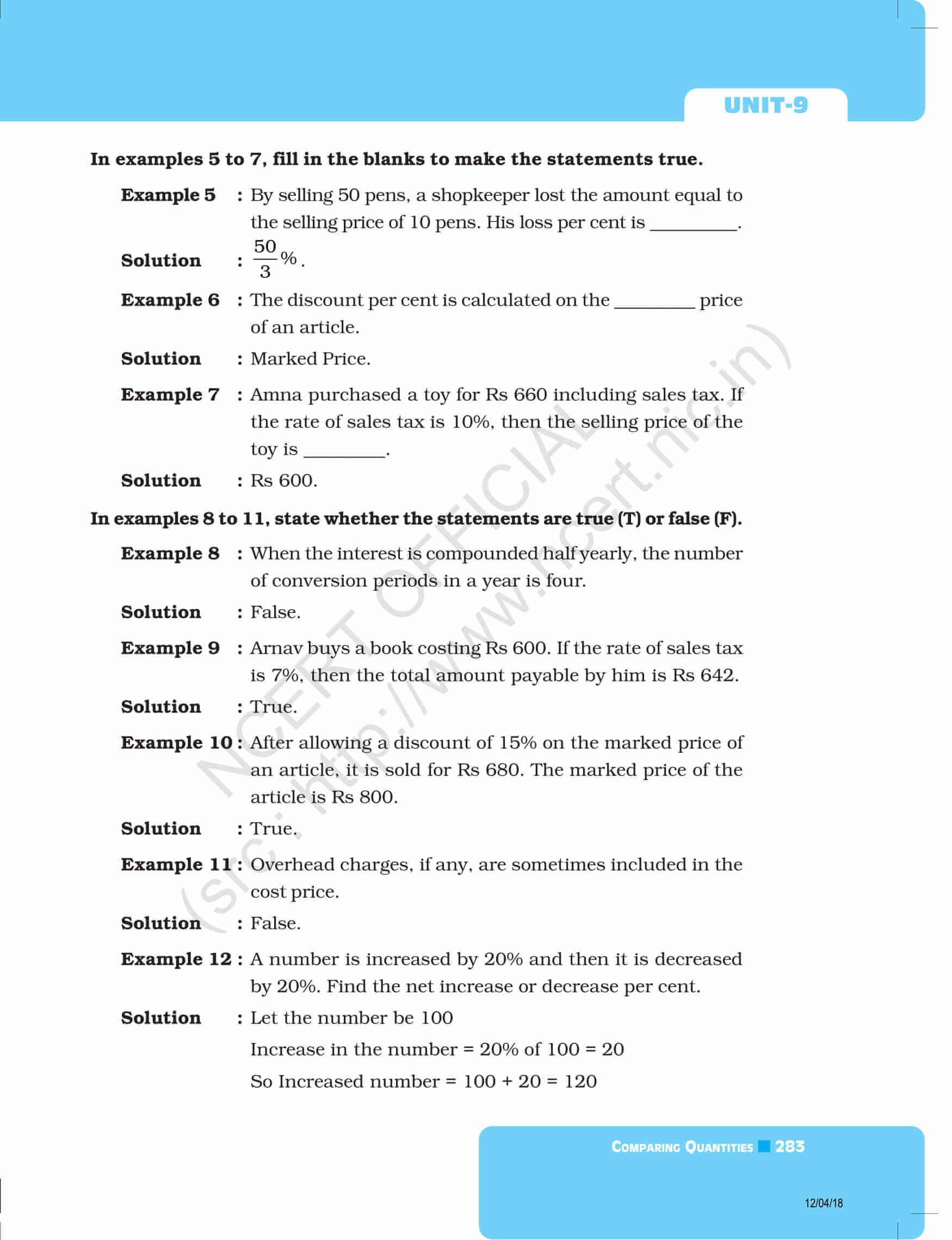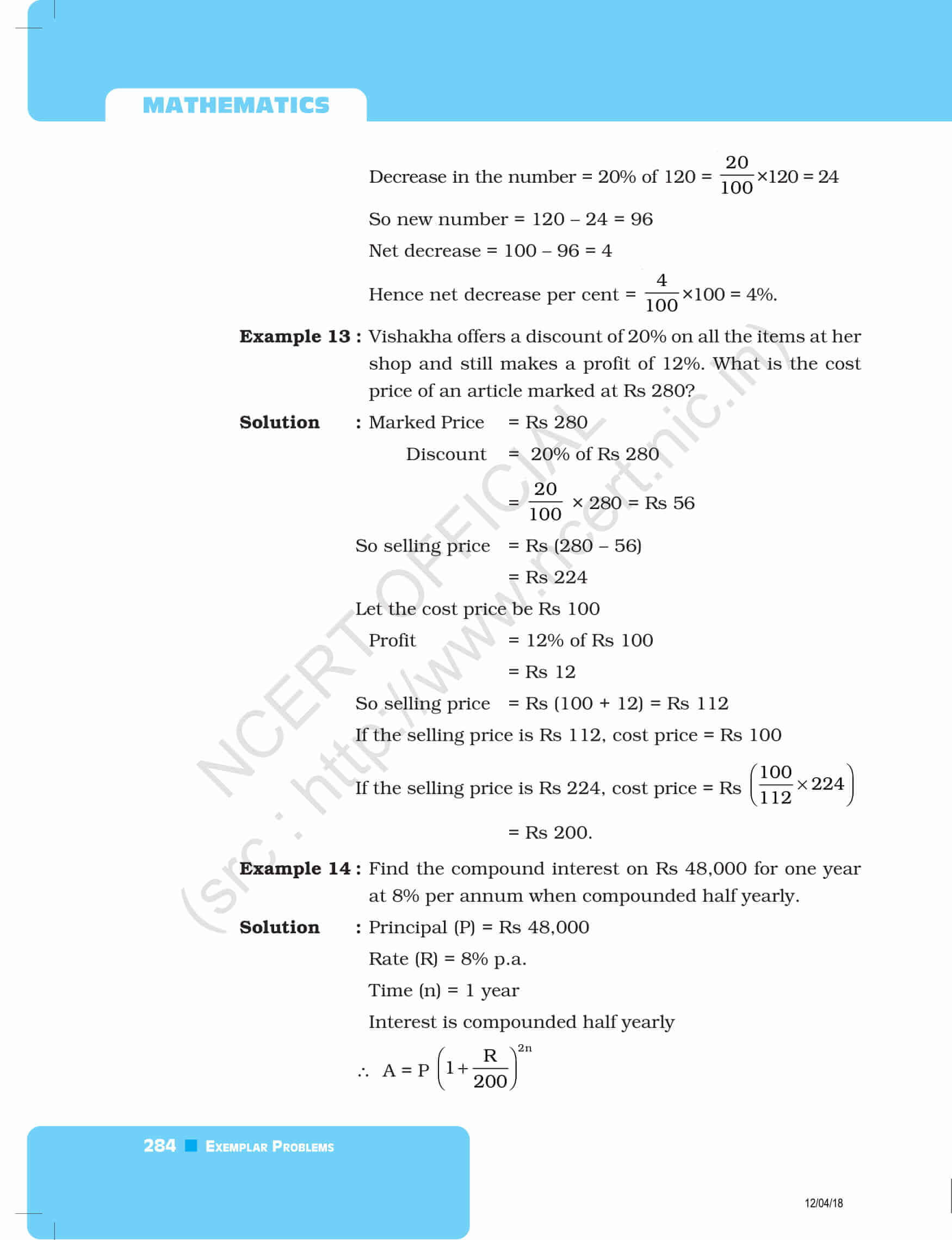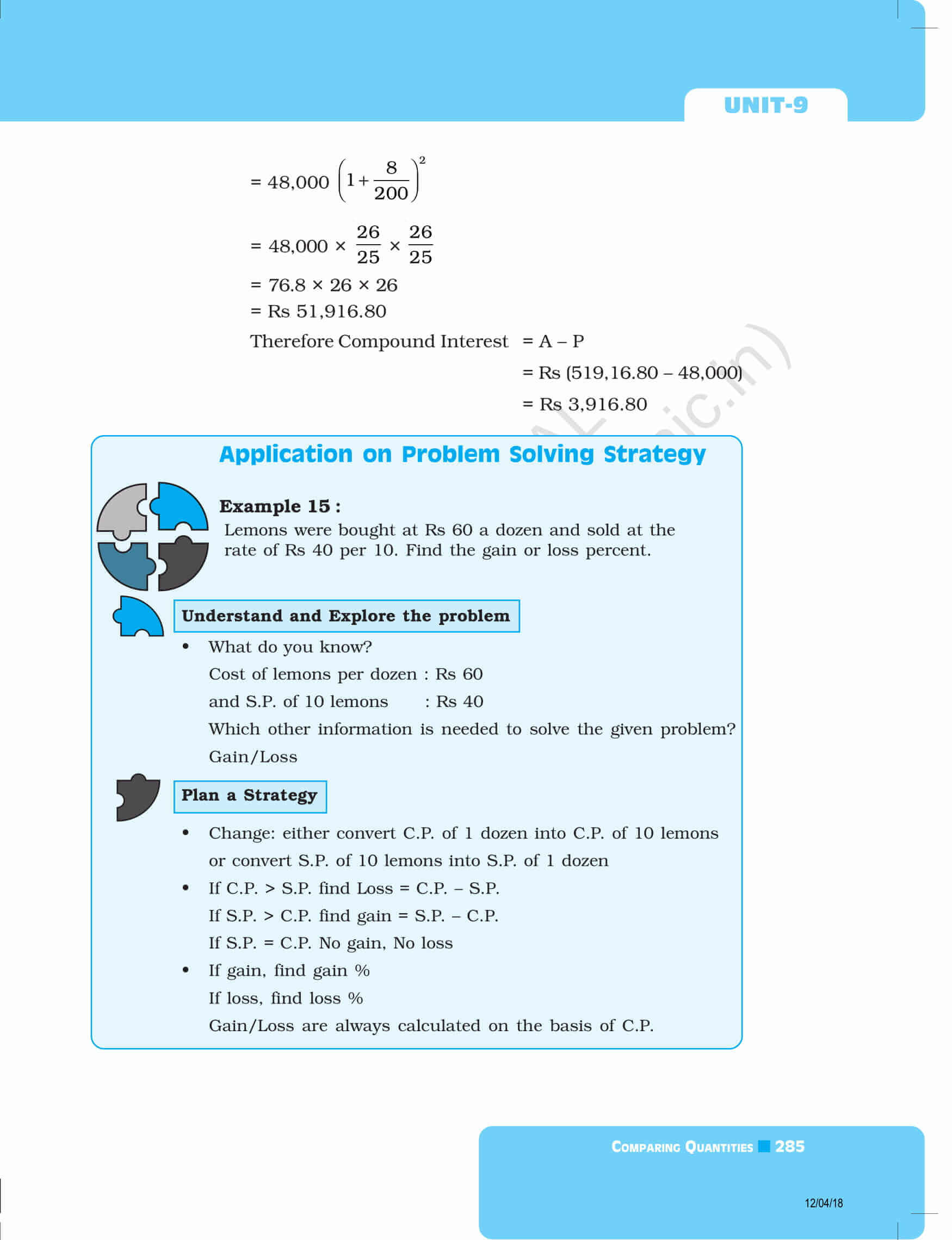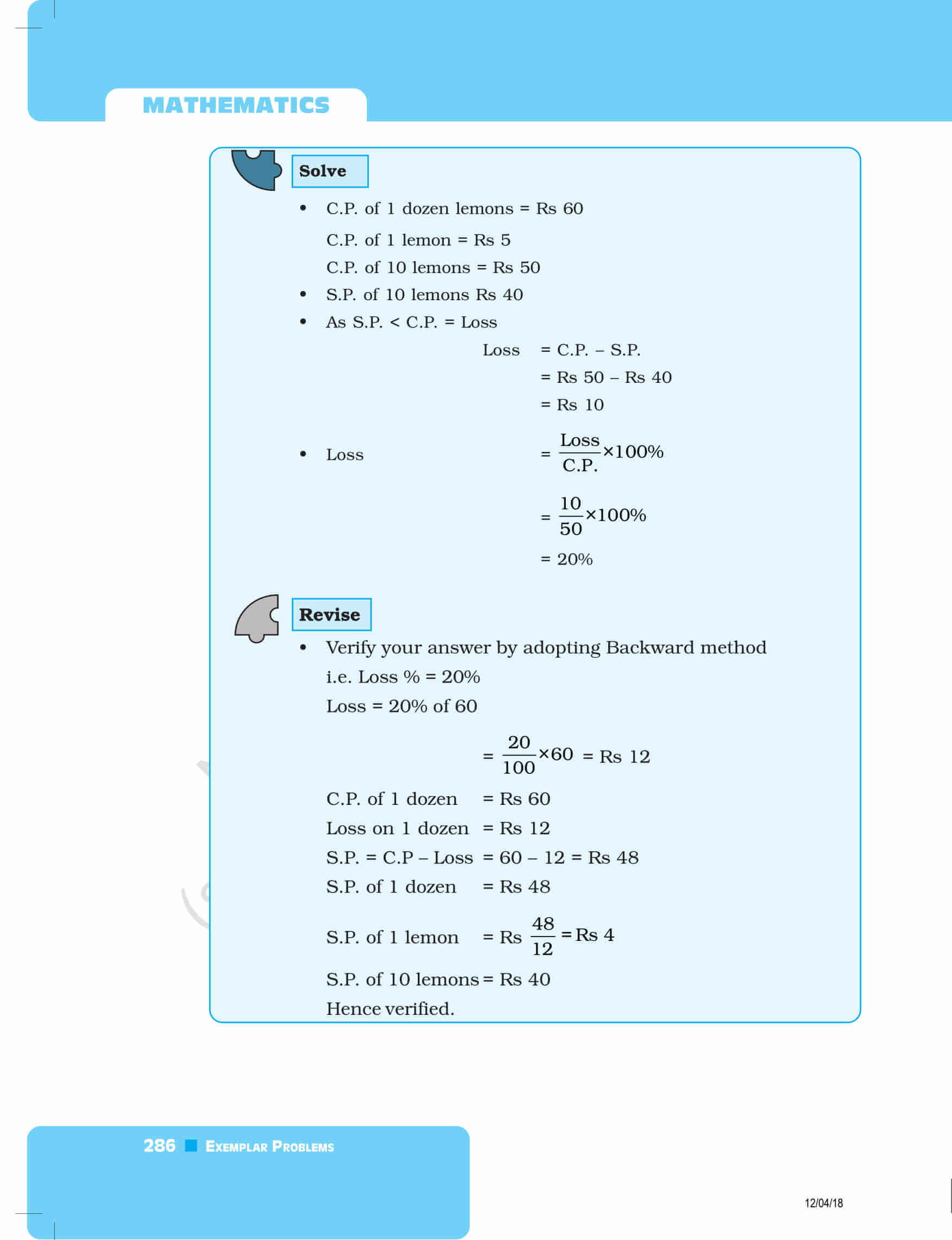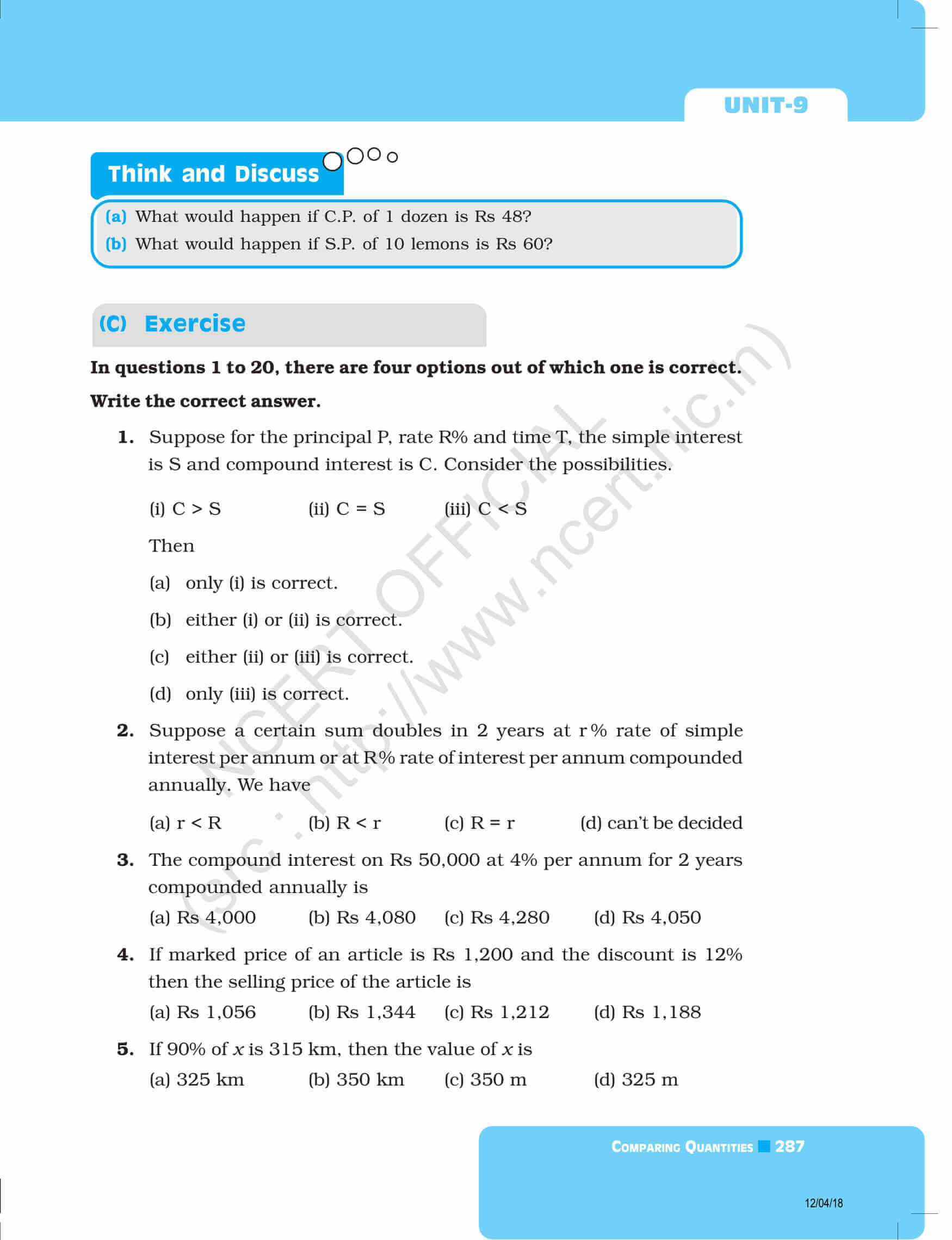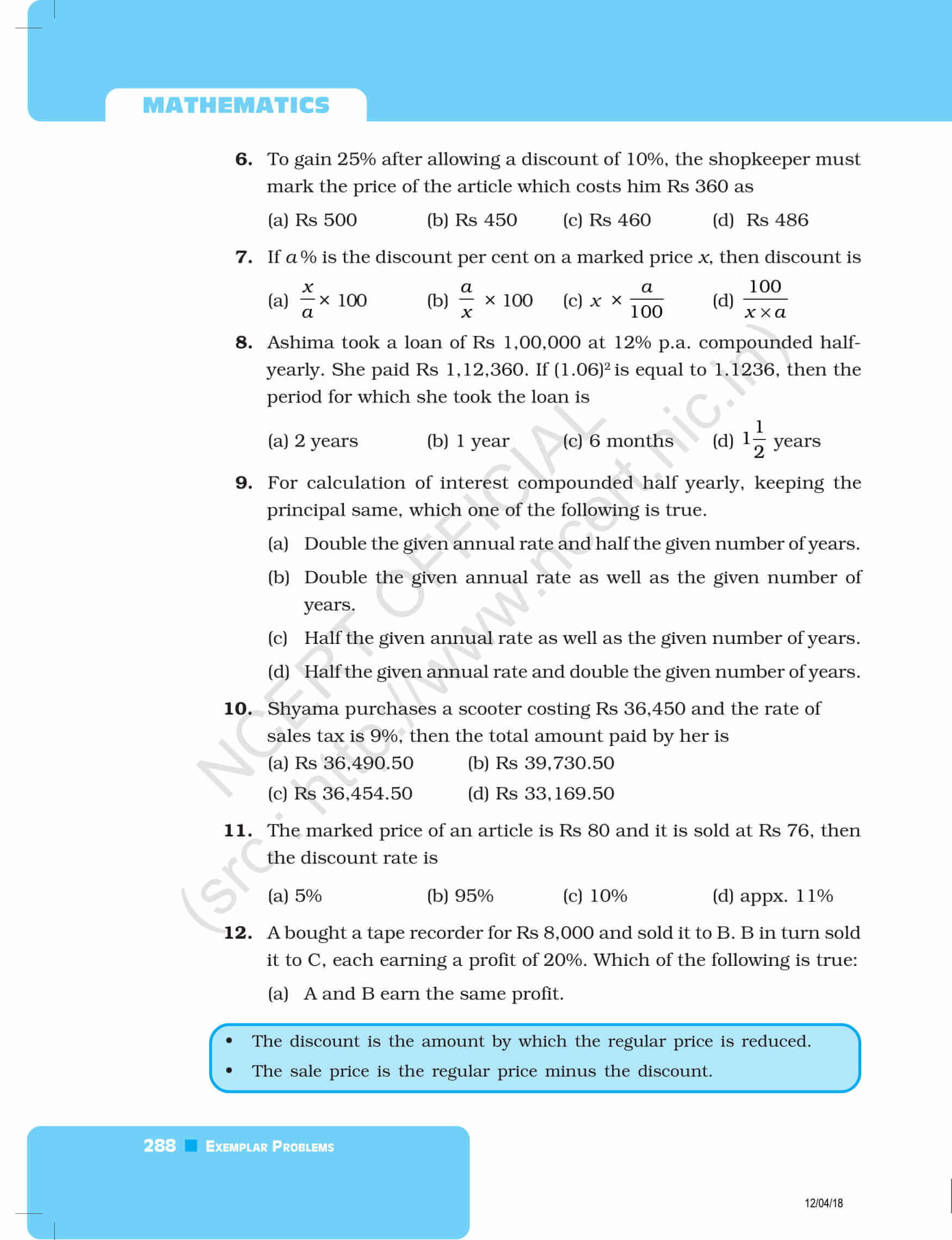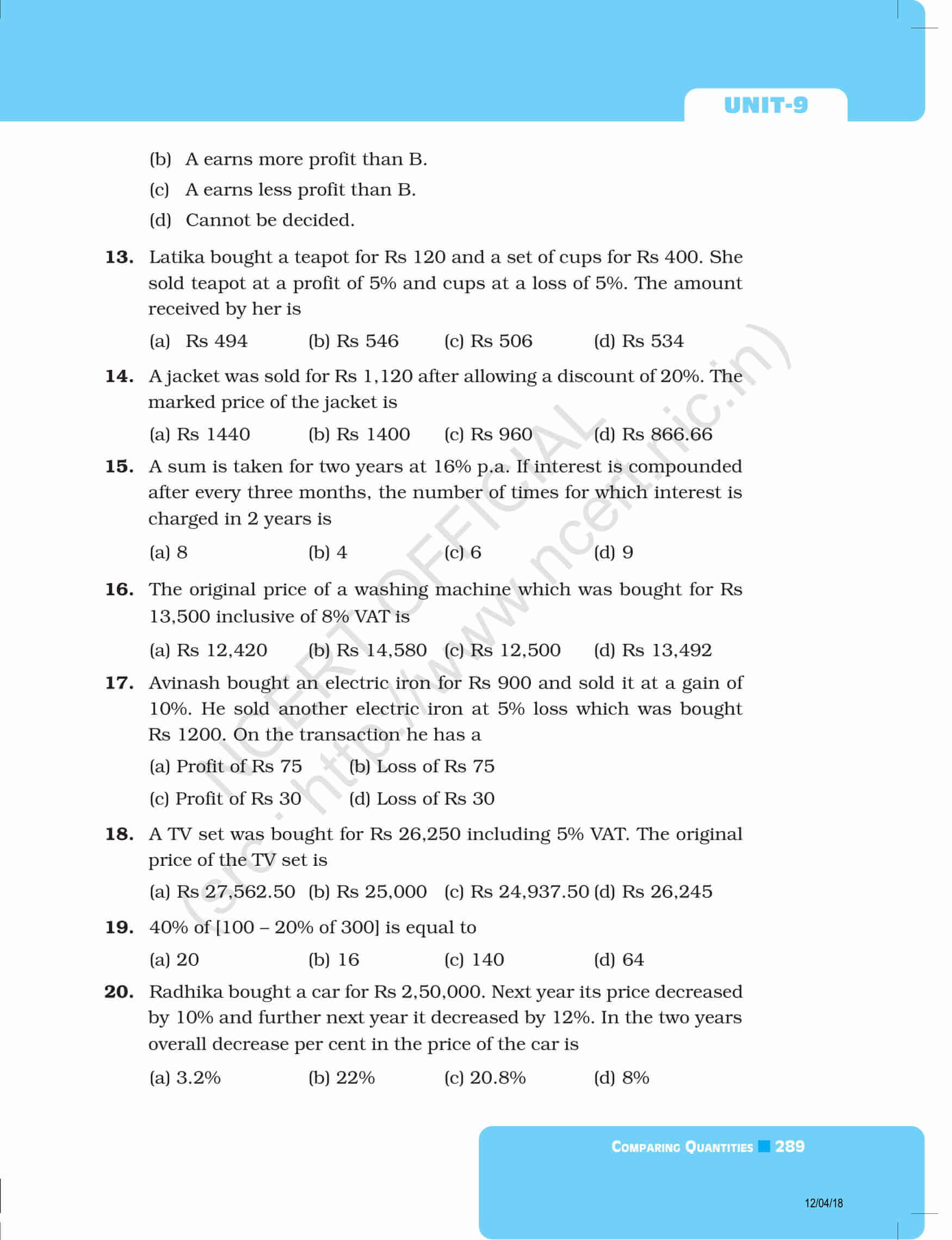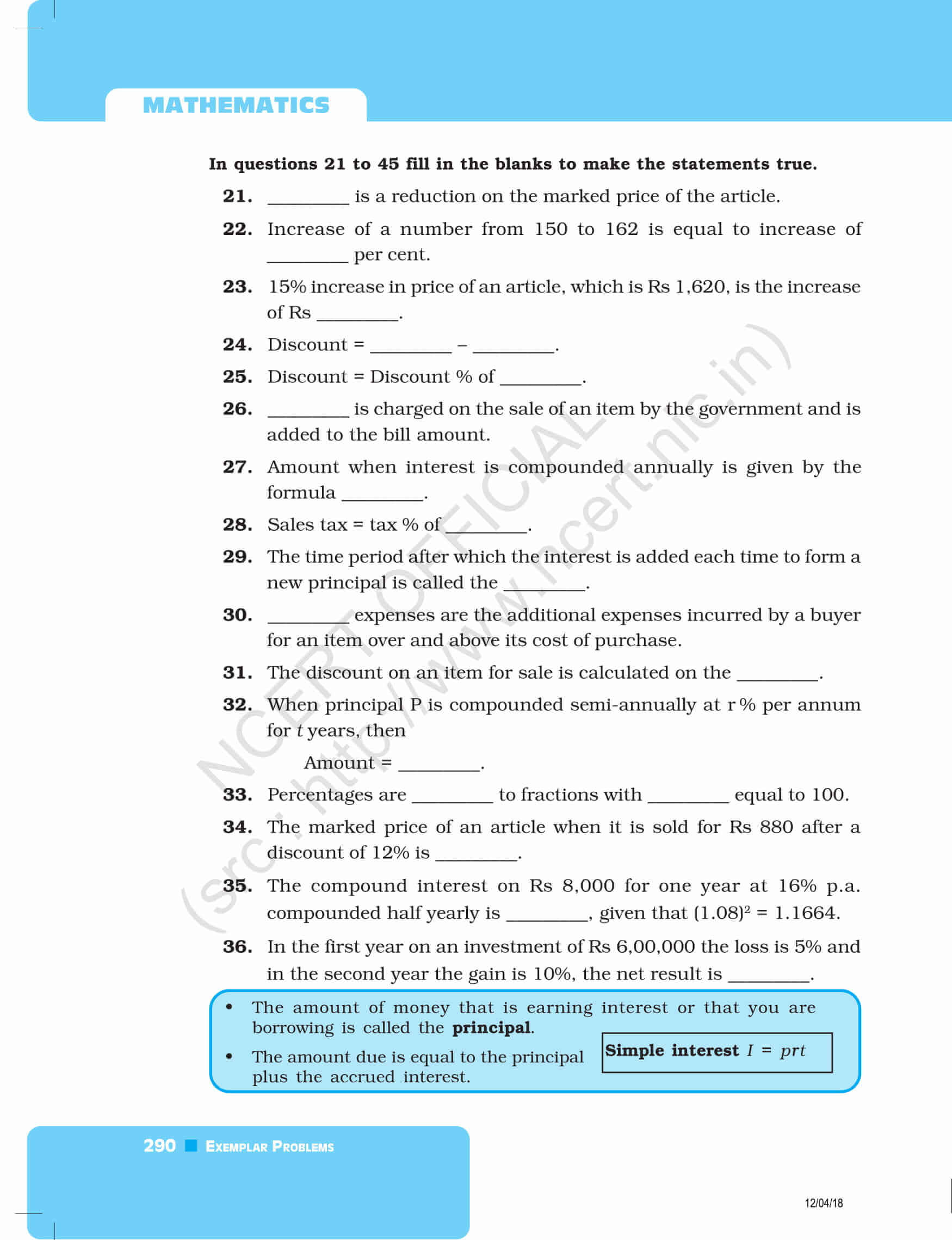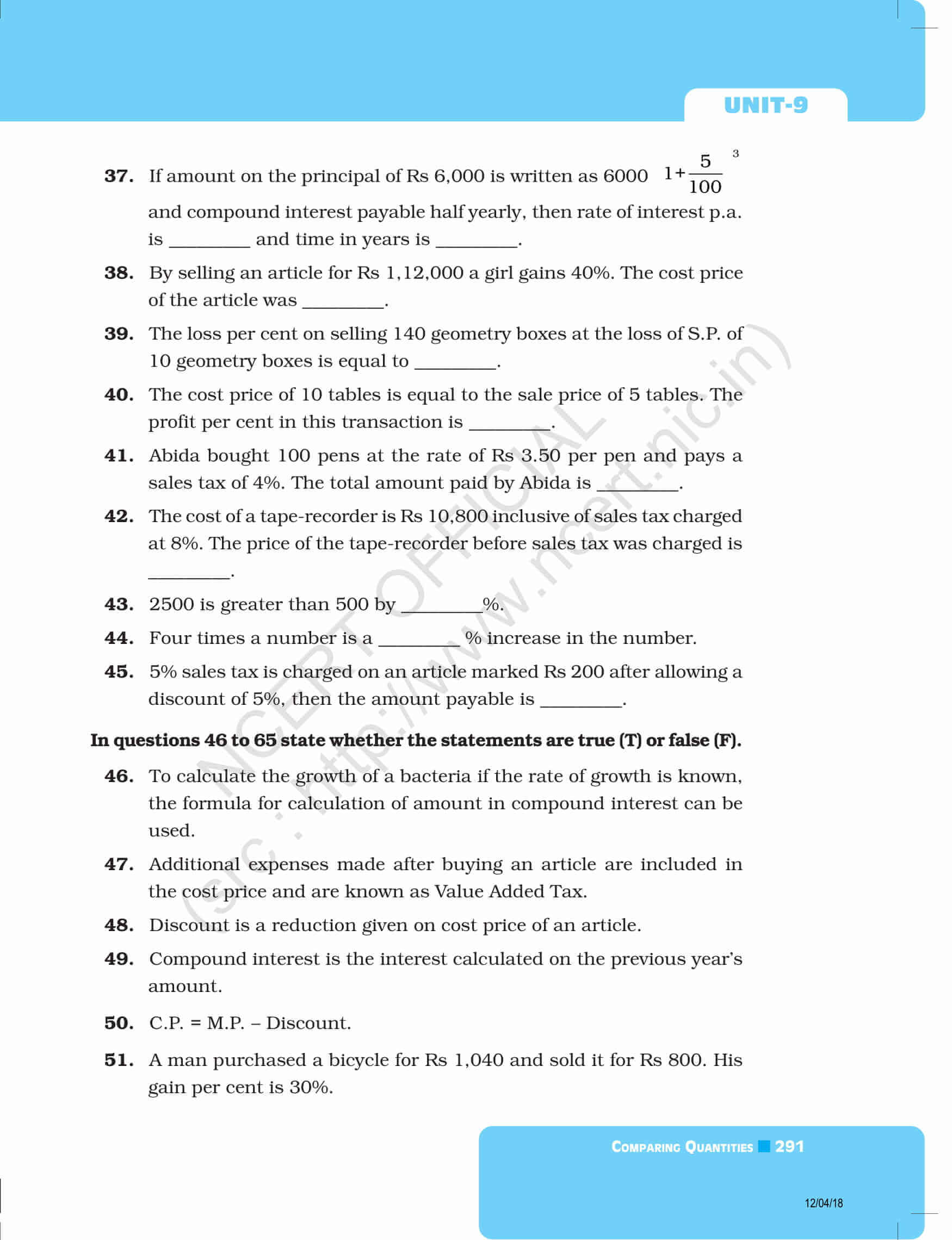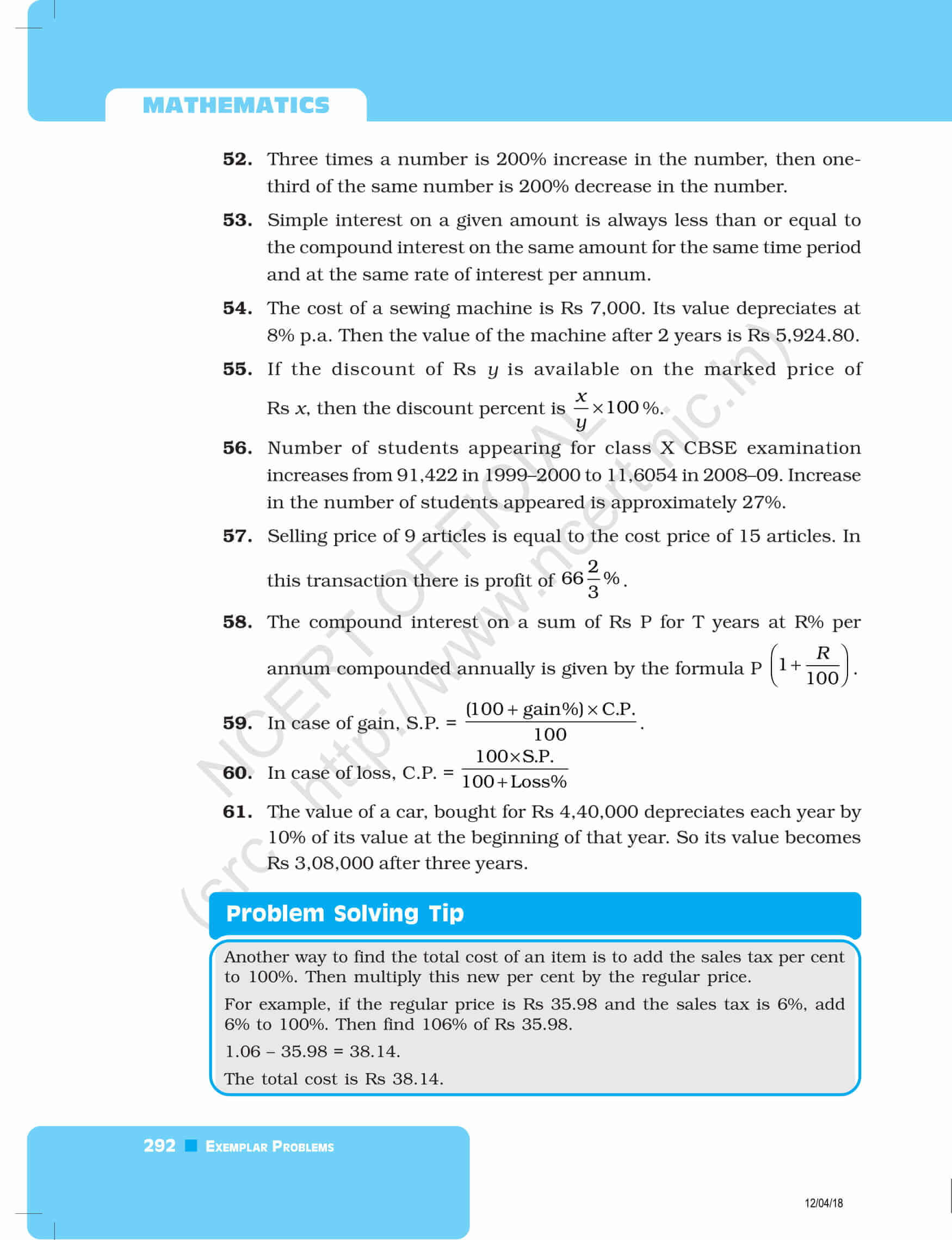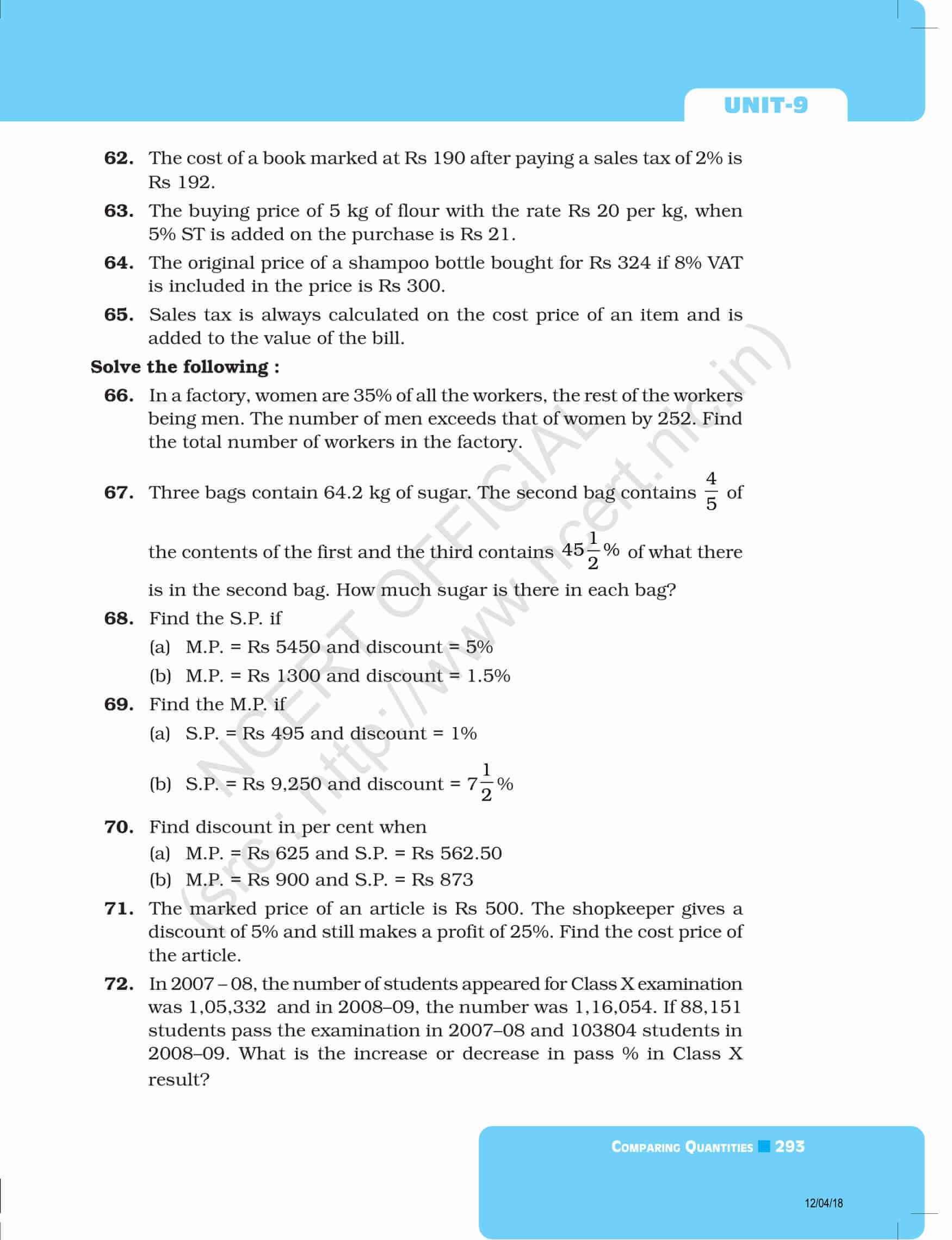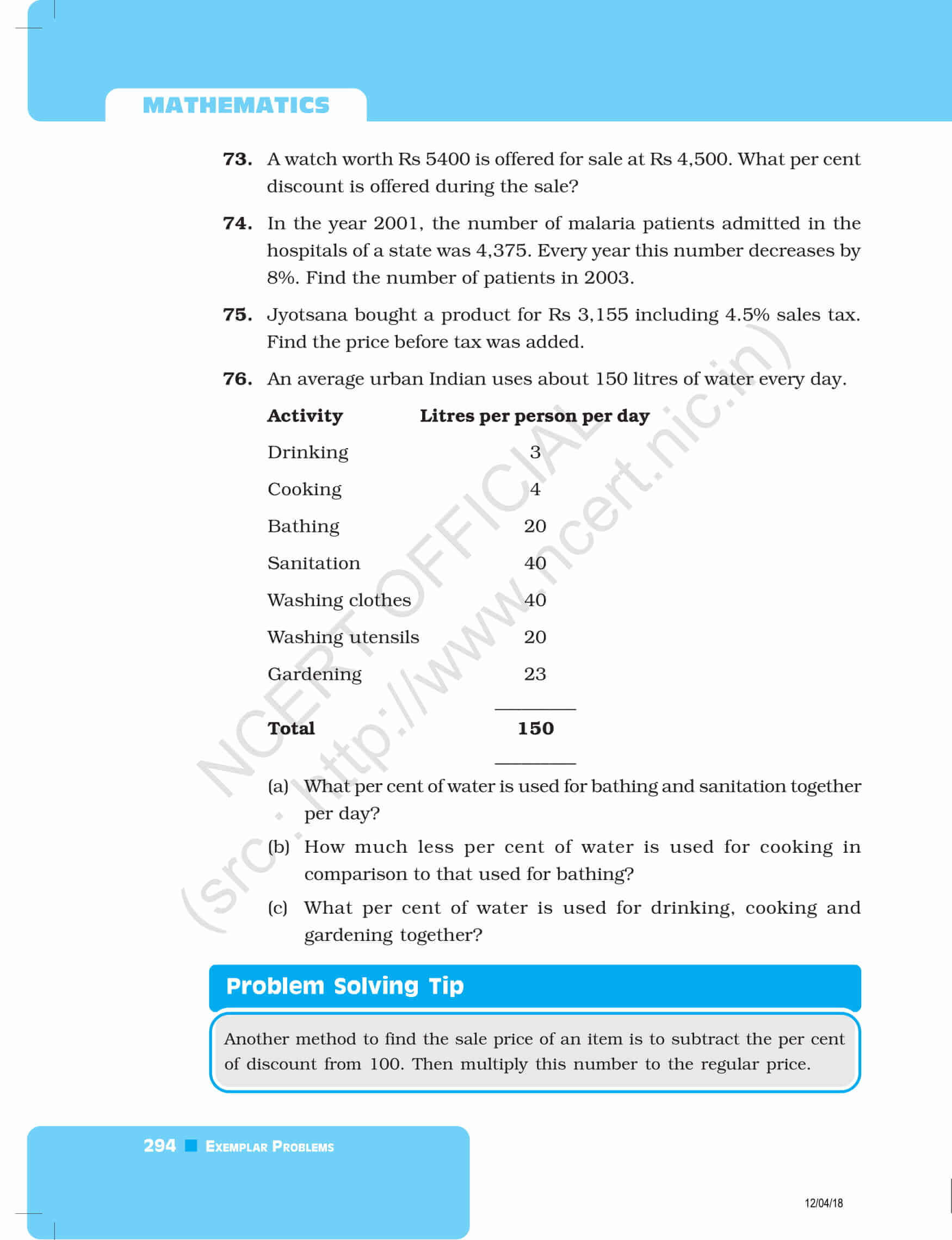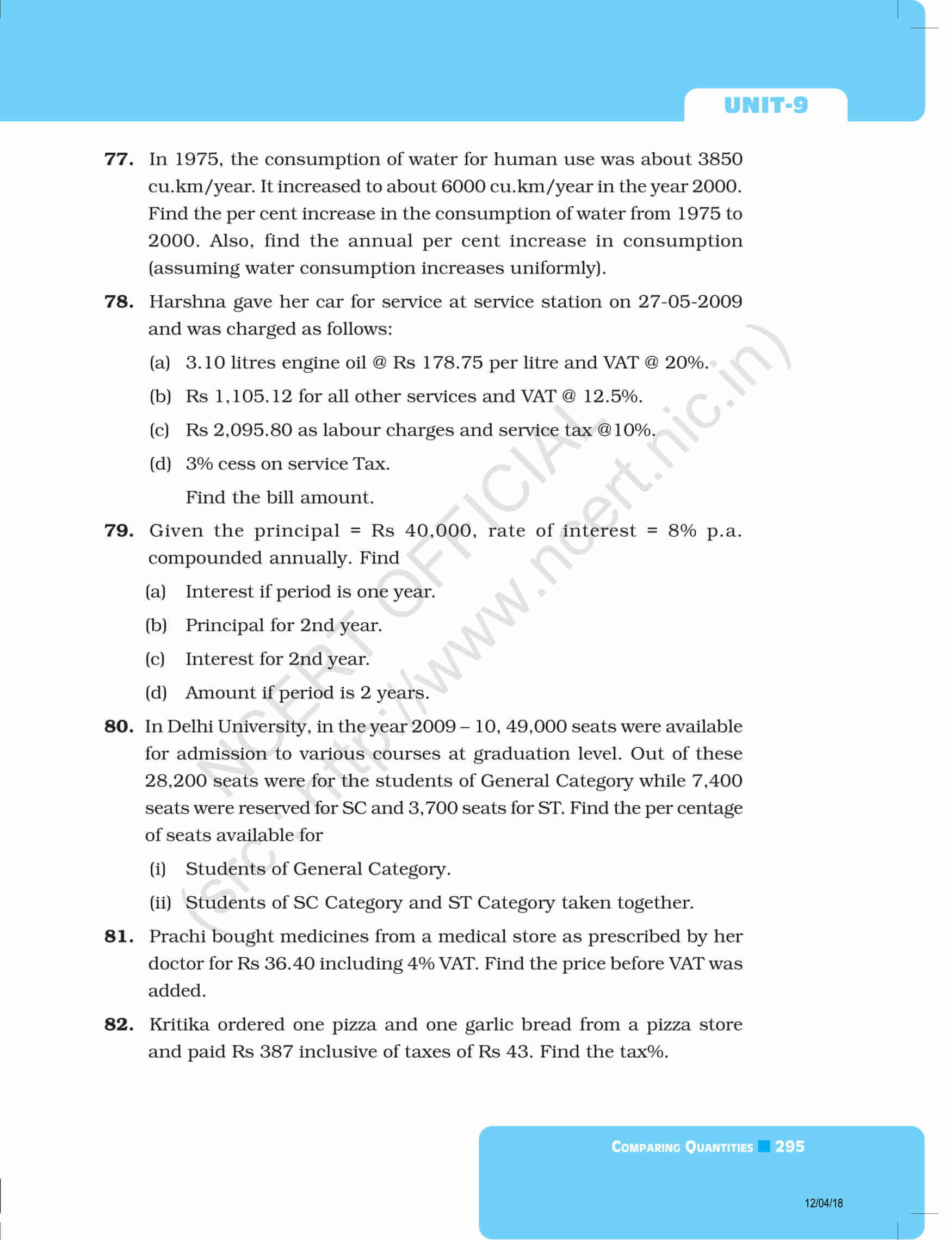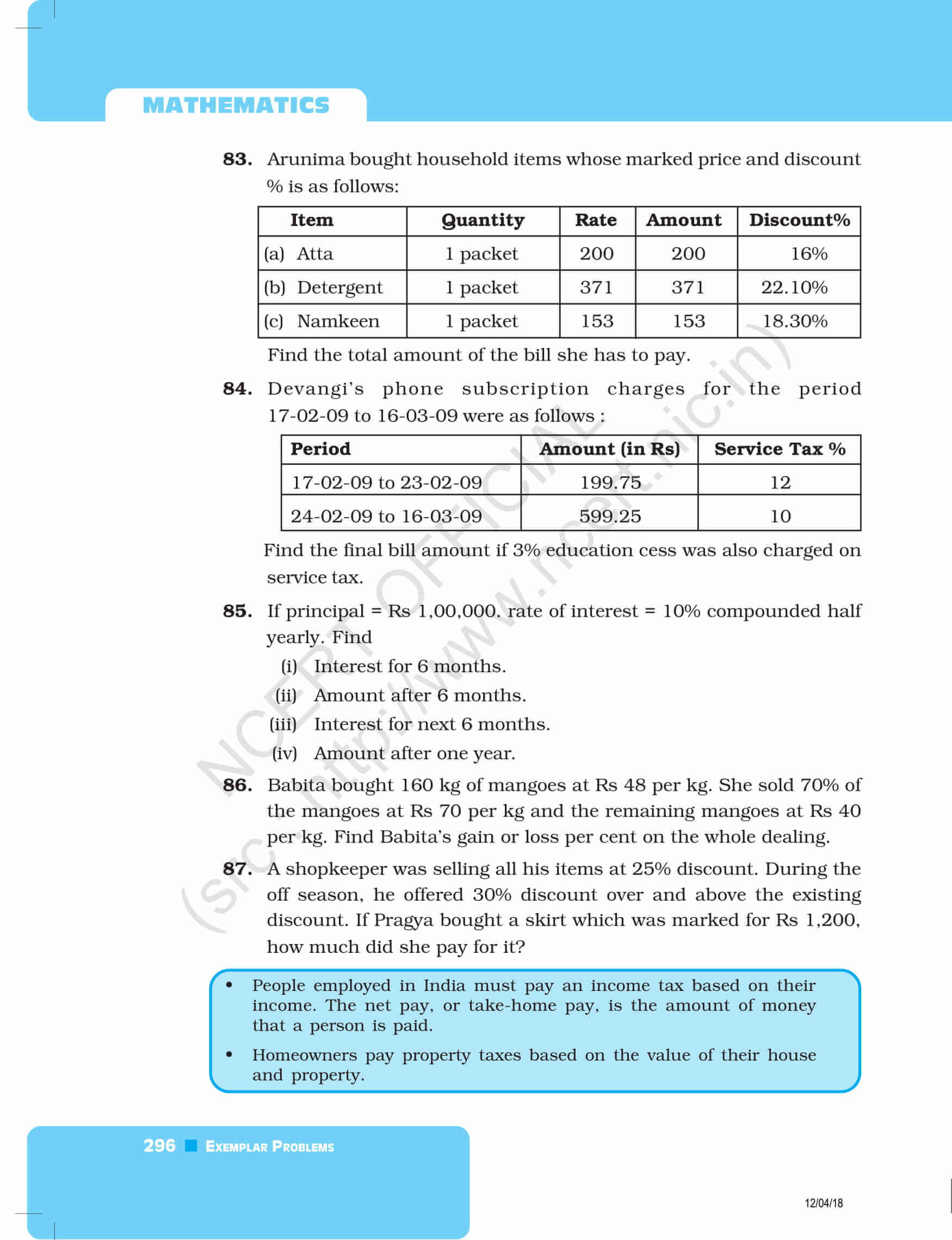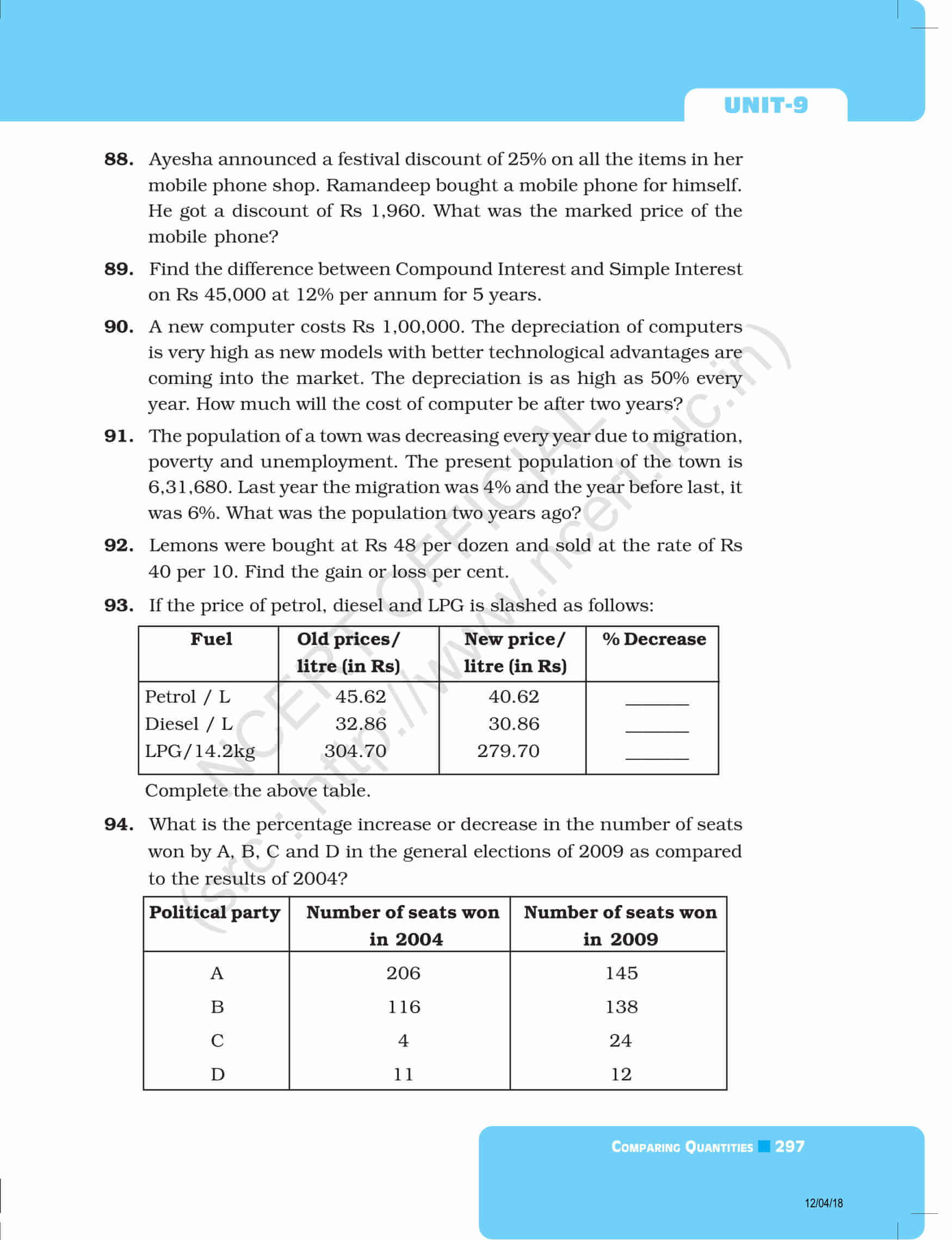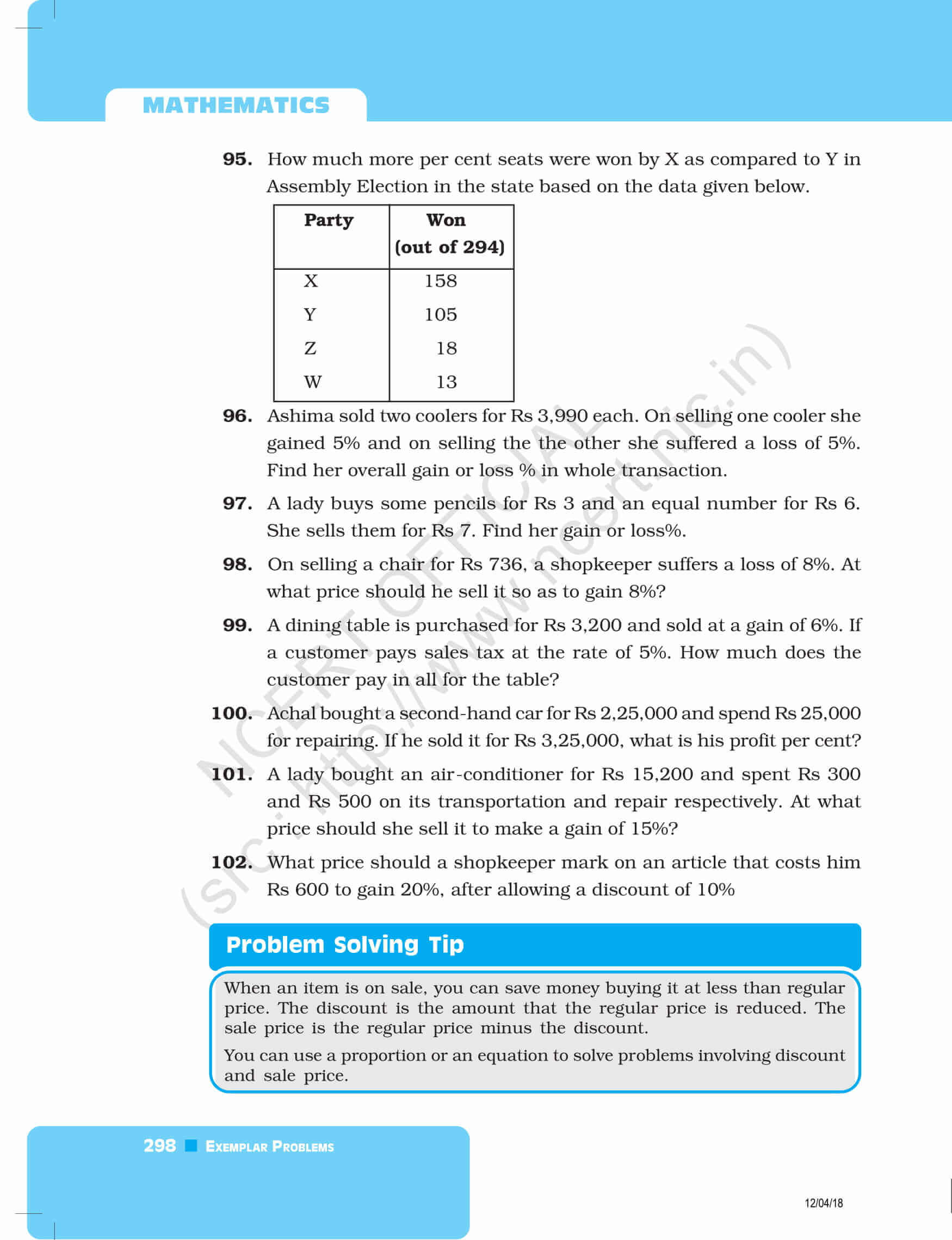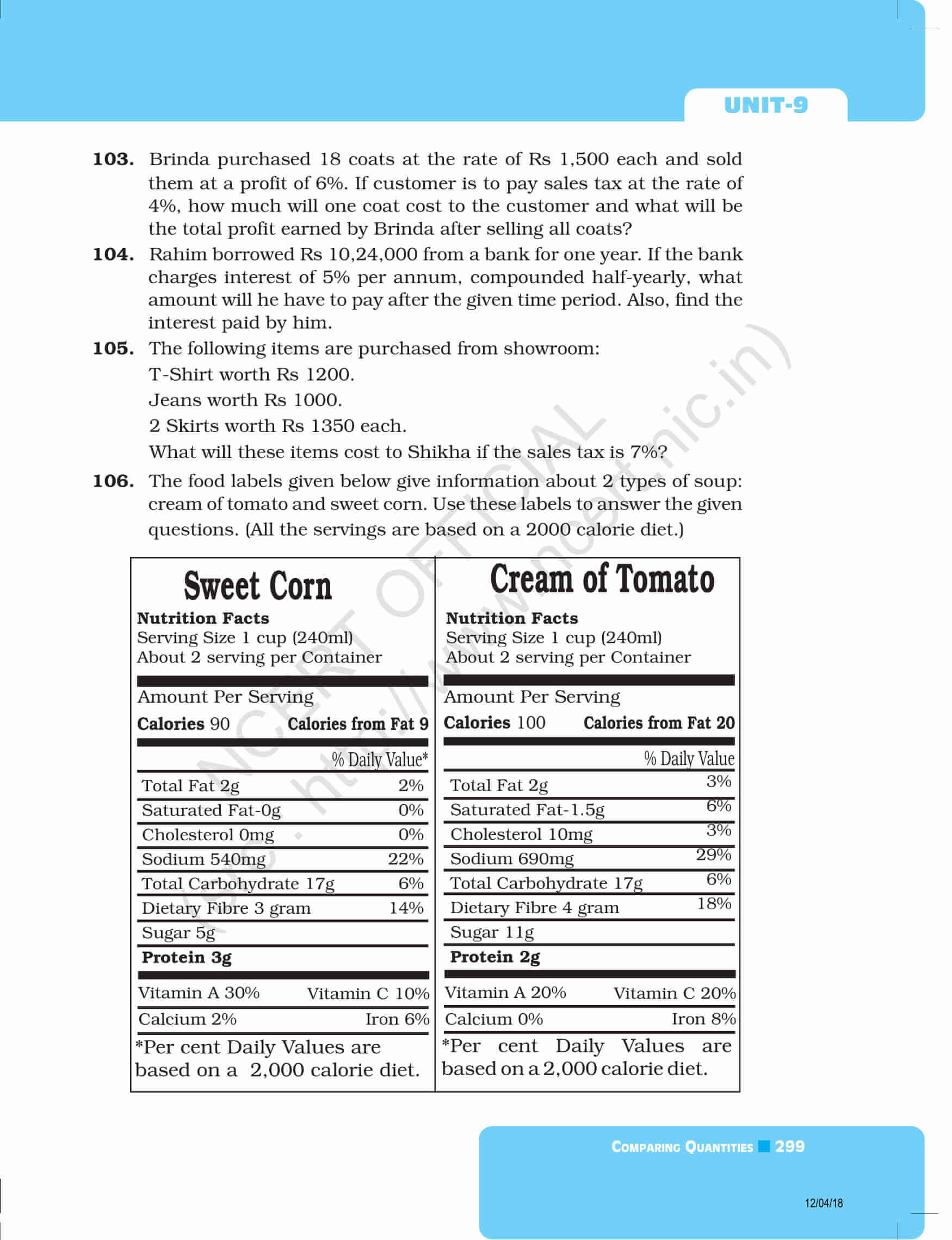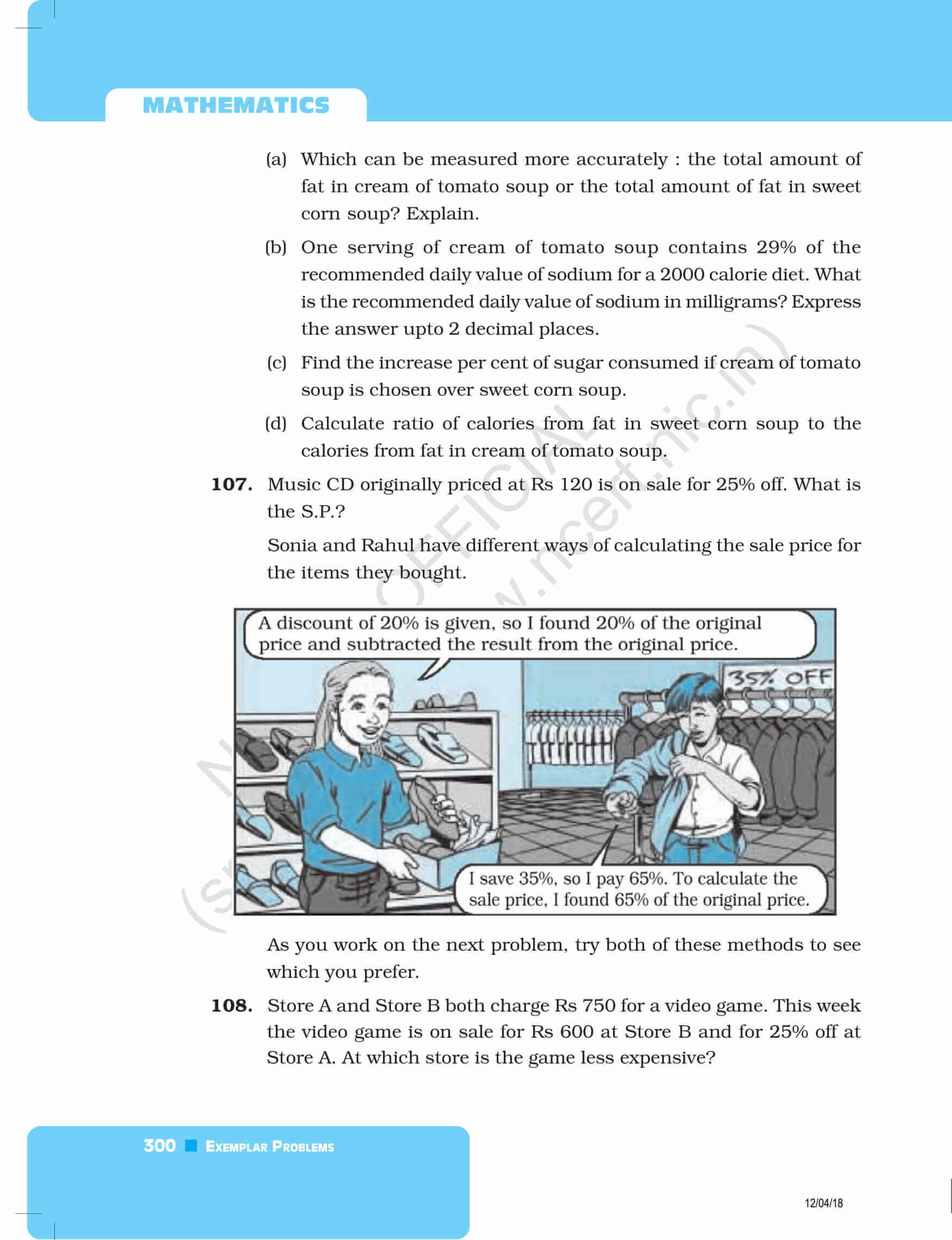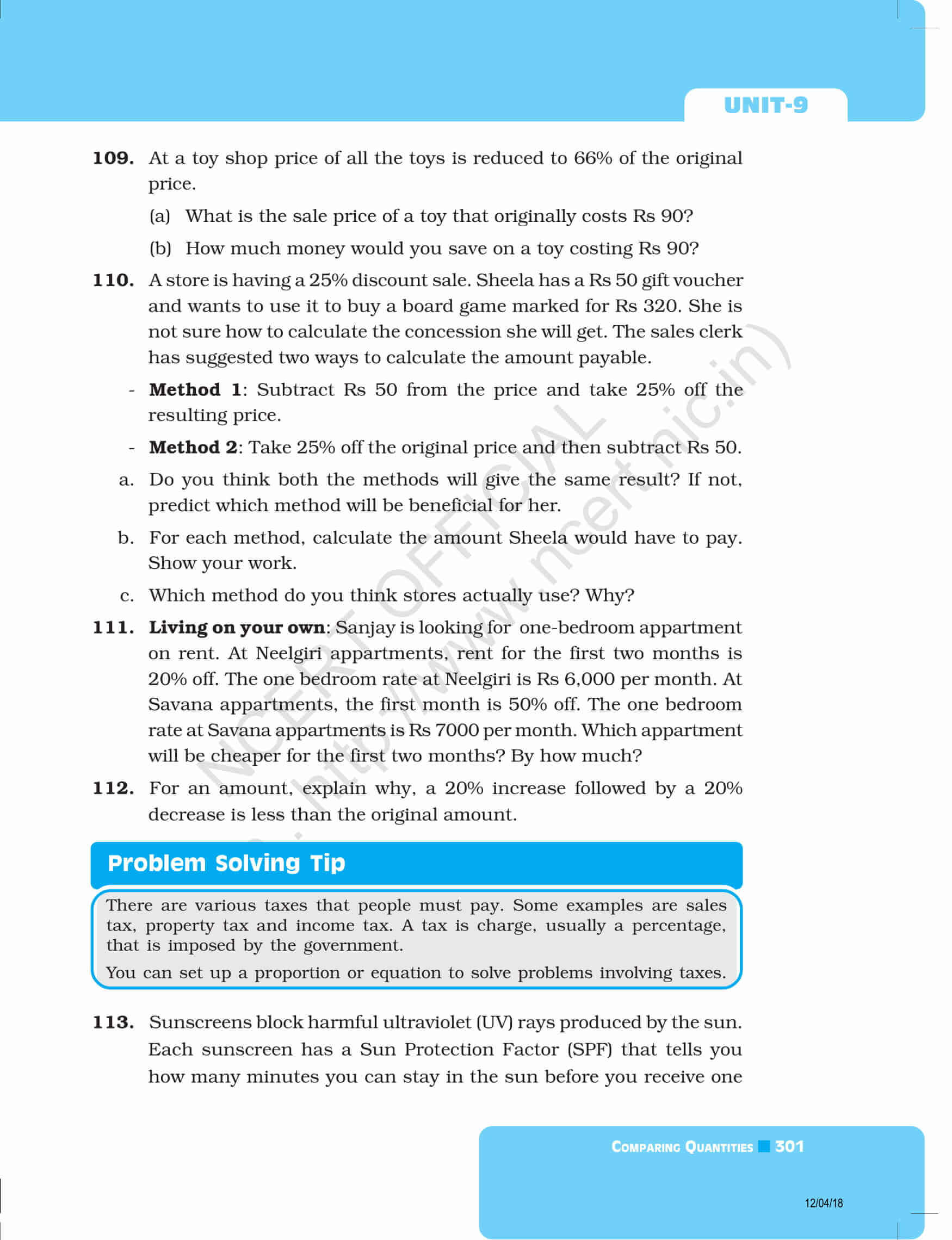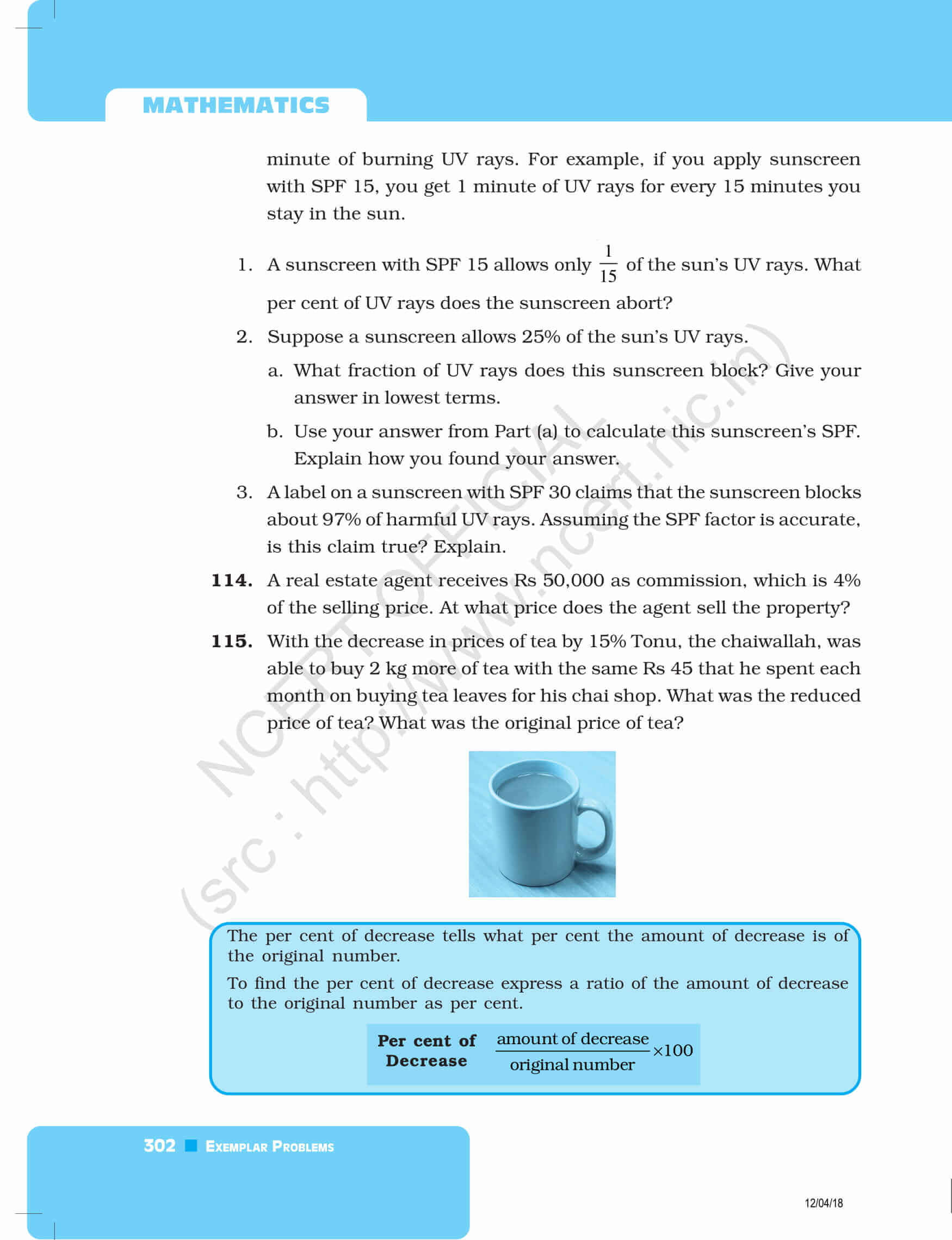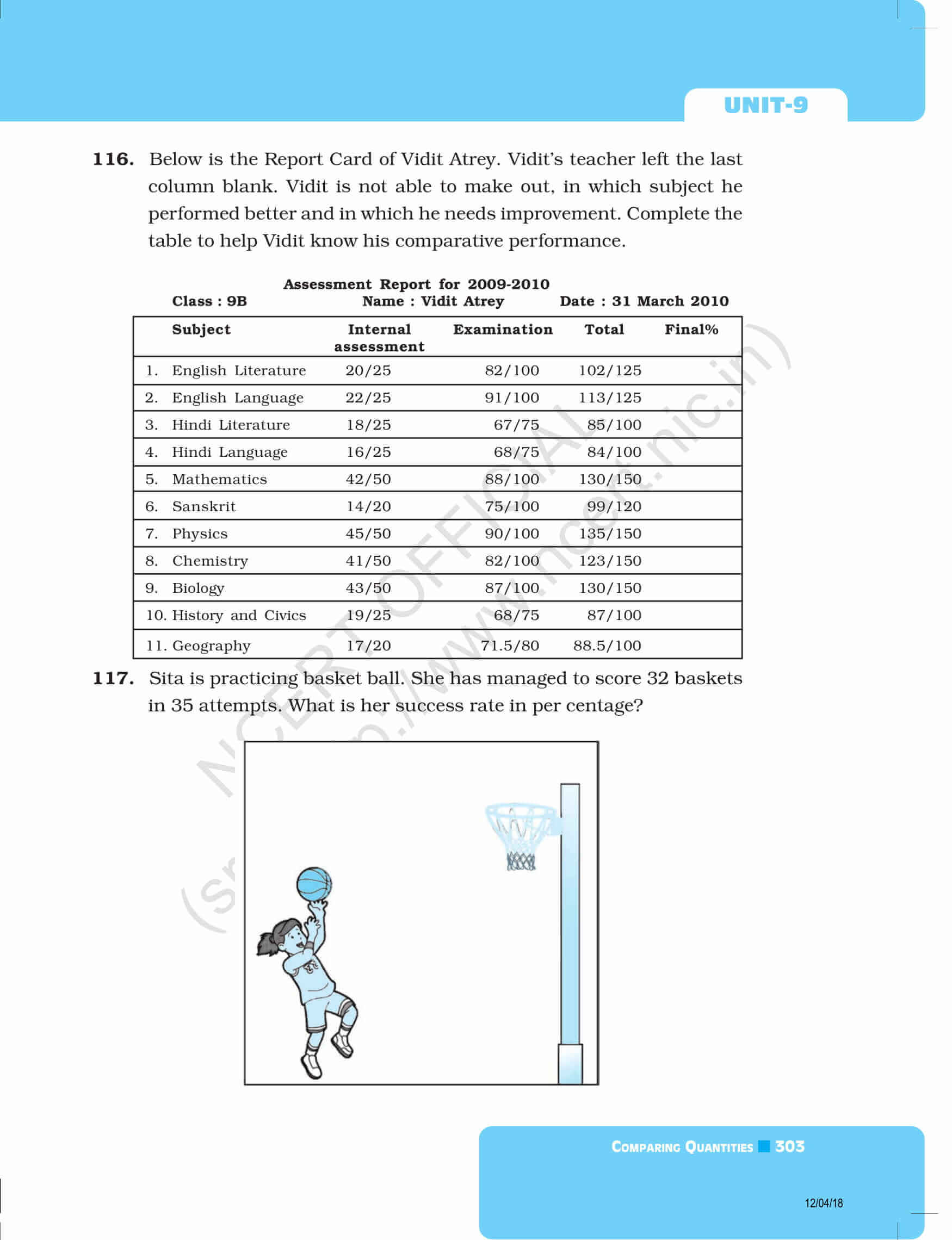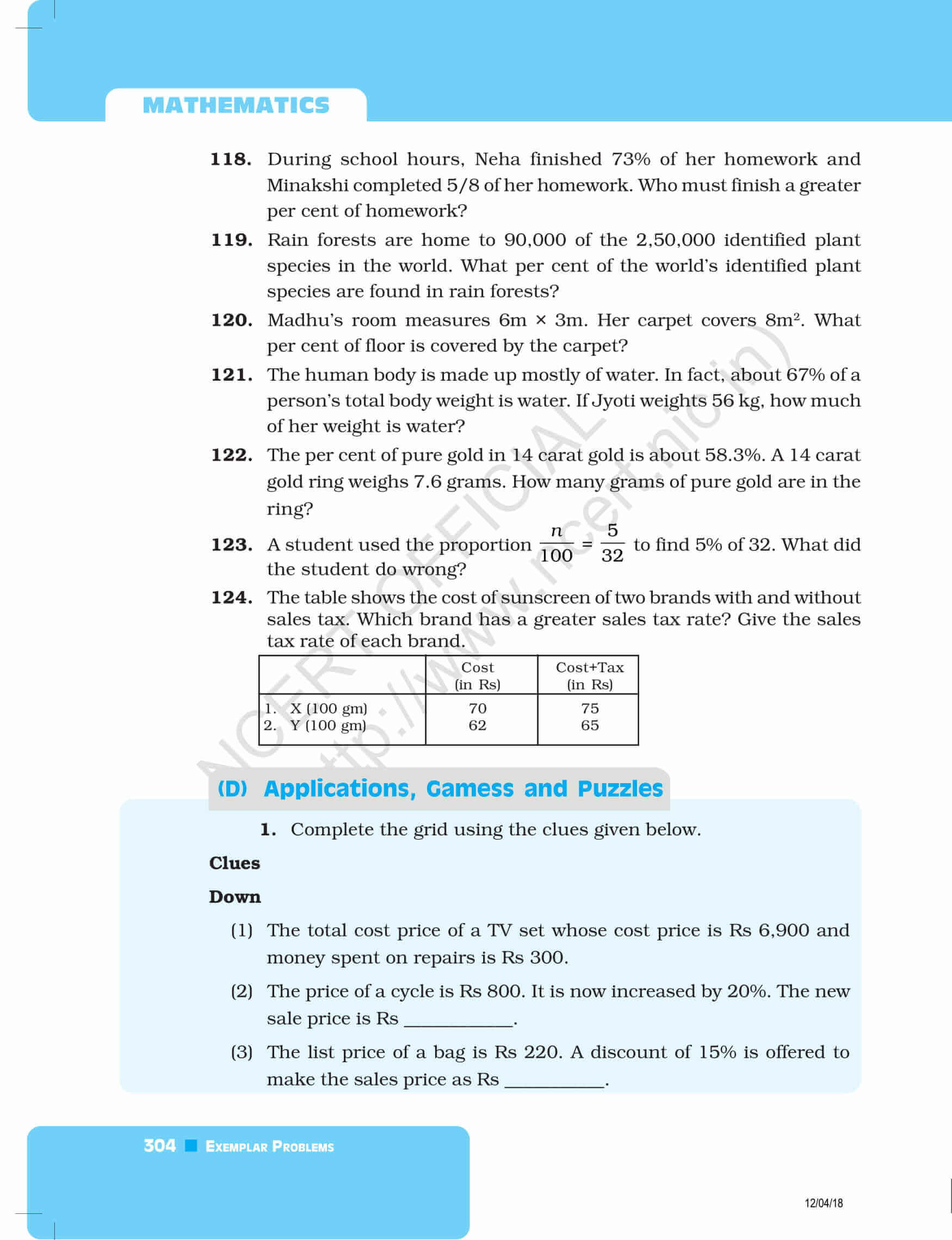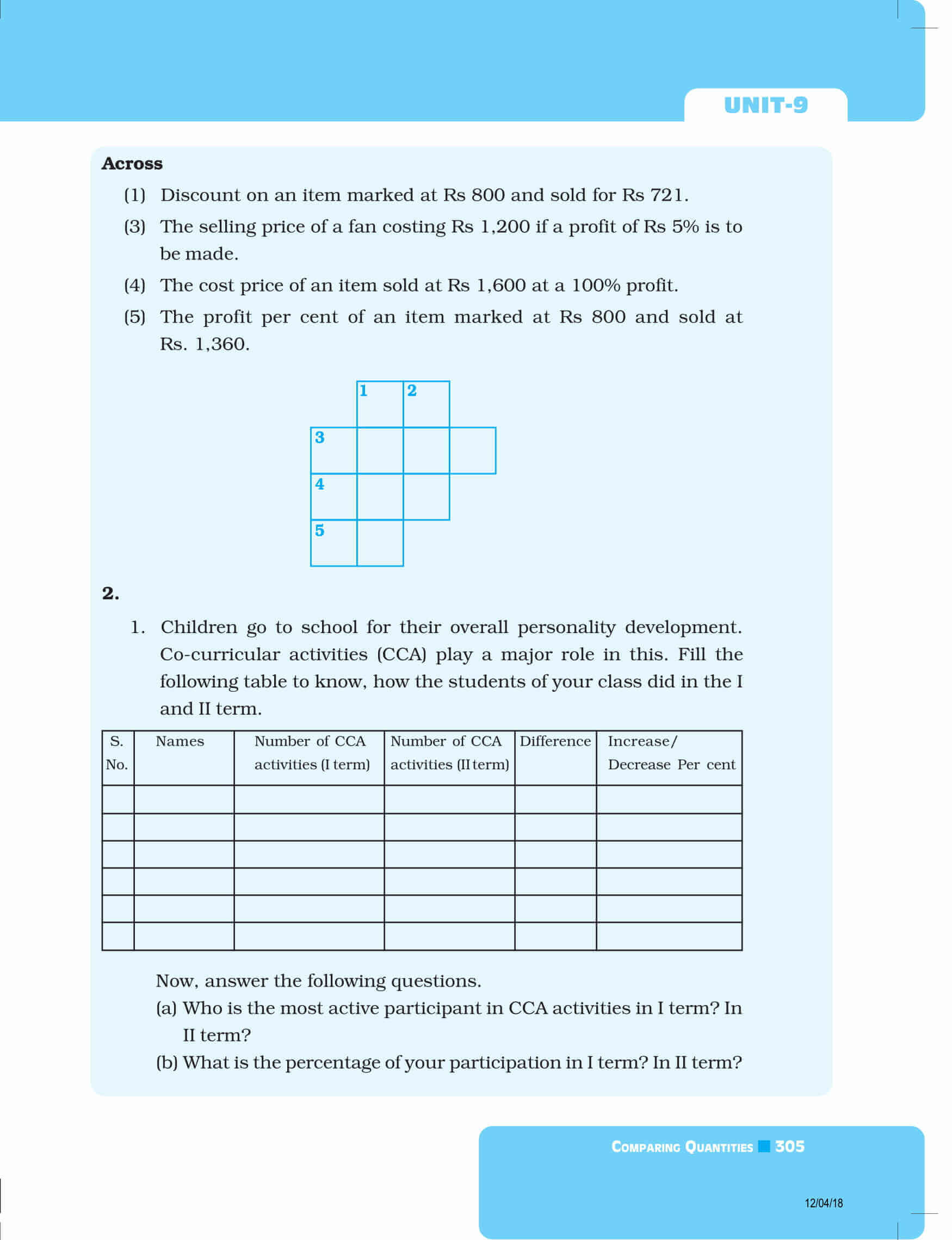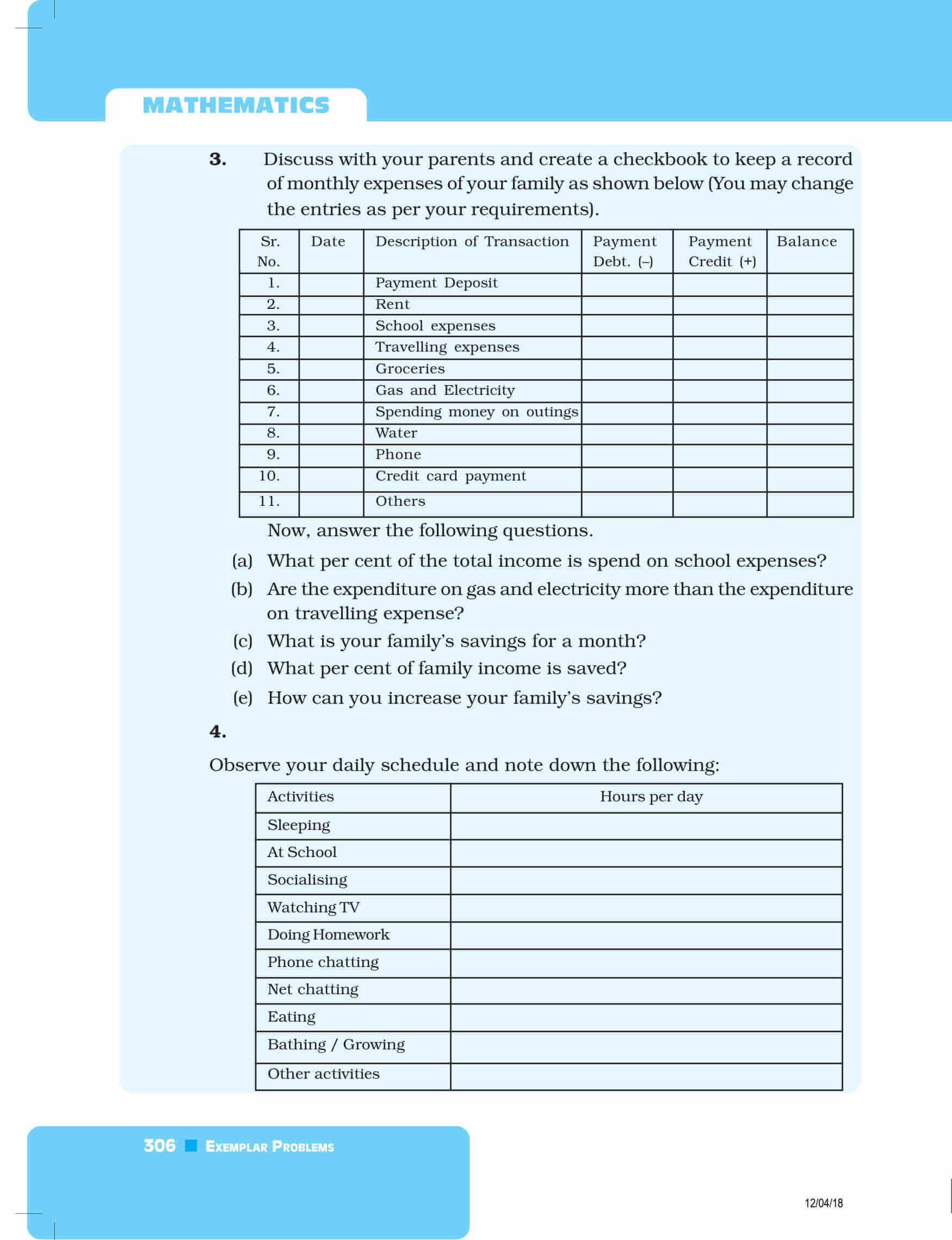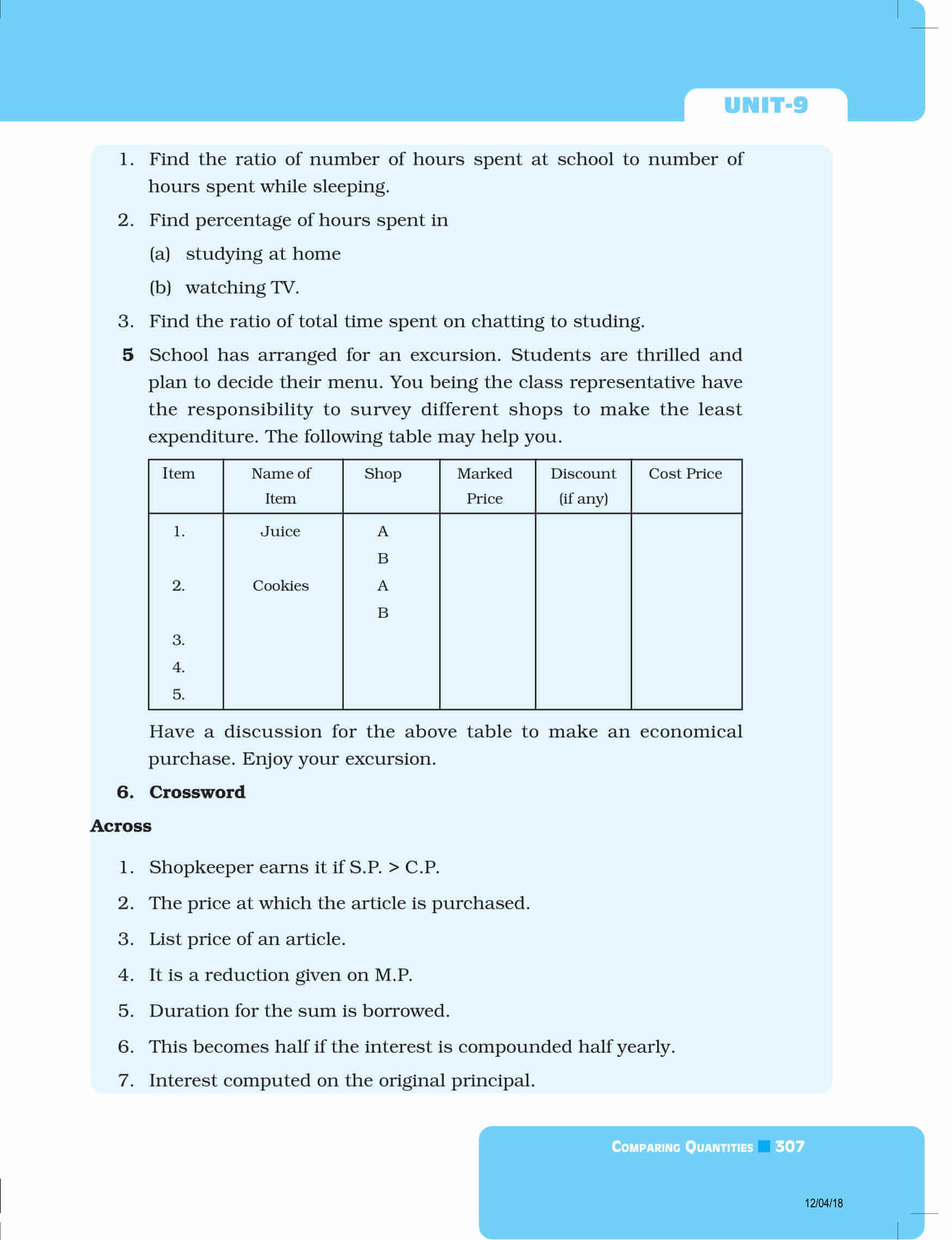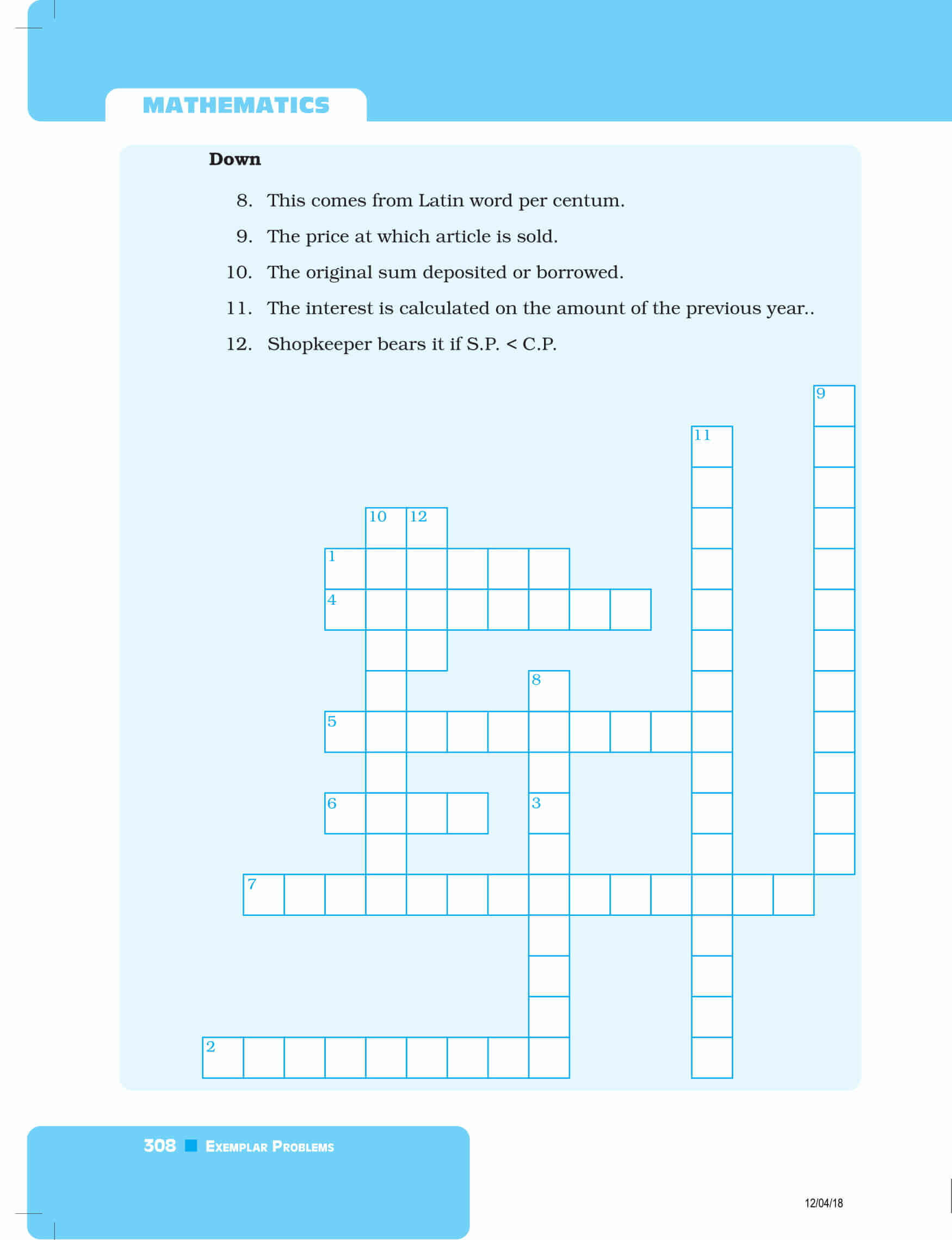Get exemplars for all chapters of Maths covered in 8th standard by clicking here. Also, download BYJU’S-The Learning App and get personalized videos and experiencing a new way of learning to understand the concepts easily.

Also Check